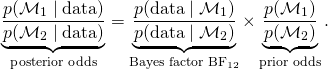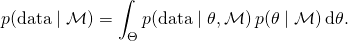# Bayes Factors for Stan Models without Tears

Bayesian inference is conceptually straightforward: we start with prior uncertainty and then use Bayes’ rule to learn from data and update our beliefs. The result of this learning process is known as posterior uncertainty. Quantities of interest can be parameters (e.g., effect size) within a single statistical model or different competing models (e.g., a regression model with three predictors vs. a regression model with four predictors). When the focus is on models, a convenient way of comparing two models M1 and M2 is to consider the model odds:In this equation, the Bayes factor quantifies the change that the data bring about in our beliefs about the relative plausibility of the two competing models (Kass & Raftery, 1995). The Bayes factor contrasts the predictive performance of M1 against that of M2, where predictions are generated from the prior distributions on the model parameters. Technically, the Bayes factor is the ratio of the marginal likelihoods of M1 and M2. The marginal likelihood of a model is the average of the likelihood of the data across all possible parameter values given that model, weighted by the prior plausibility of those parameter values:Although straightforward in theory, the practical computation of this innocent-looking integral can be extremely challenging. As it turns out, the integral can be evaluated “by hand” for only a limited number of relatively simple models. For models with many parameters and a hierarchical structure, this is usually impossible, and one needs to resort to methods designed to estimate this integral instead.

In our lab, we regularly apply a series of hierarchical models and we often wish to compare their predictive adequacy. For many years we have searched for a reliable and generally applicable method to estimate the innocent-looking integral. Finally, we realized that what we needed was professional help.Figure 1. The Koosje note: Jon Forster’s original lunchtime explanation of bridge sampling.

### Bridge Sampling to the Rescue

Professional help came in the form of Prof. Jon Forster, who, while enjoying lunch in Koosje, a typical Amsterdam cafe, scribbled down the key equations that define bridge sampling (Meng & Wong, 1996; see Figure 1 for historical evidence). “Why don’t you just use this”, Jon said, “it is easy and reliable”. And indeed, after implementing the Koosje note, we were struck by the performance of the bridge sampling methodology. In our experience, the procedure yields accurate and reliable estimates of the marginal likelihood — also for hierarchical models. For details on the method, we recommend our recent tutorial on bridge sampling and our paper in which we apply bridge sampling to hierarchical Multinomial Processing Tree models.

### bridgesampling R Package

One remaining challenge with bridge sampling is that it may be non-trivial for applied researchers to implement from scratch. To facilitate the application of the method, together with Henrik Singmann we implemented the bridge sampling procedure in the bridgesampling R package. The bridgesampling package is available from CRAN and the development version can be found on Github1. For models fitted in Stan (Carpenter et al., 2017) using rstan (Stan Development Team, 2017) the estimation of the marginal likelihood is automatic: one only needs to pass the stanfit object to the bridge_sampler function which then produces an estimate of the marginal likelihood. Note that the models need to be implemented in a way that retains all constants. However, this is fairly easy to achieve and is described in detail in our paper about the bridgesampling package.

With the marginal likelihood estimate in hand one can compare models using Bayes factors or posterior model probabilities; or one can combine different models using Bayesian model averaging. In our paper about the package, we show how to apply bridge sampling to Stan models with a generalized linear mixed model (GLMM) example and a Bayesian factor analysis example where one is interested in inferring the number of relevant latent factors. Code to reproduce the examples is included in the paper and in the R package itself (which also includes further examples and vignettes), and can also be found on the Open Science Framework.

### Take-Home Message

The bridgesampling package facilitates the computation of the marginal likelihood for a wide range of different statistical models. For models implemented in Stan (such that the constants are retained), executing the code bridge_sampler(stanfit) automatically produces an estimate of the marginal likelihood.

## Like this post?

#### Footnotes

1 That is, the package can be installed in R from CRAN via install.packages("bridgesampling"). The development version can be installed from Github via devtools::install_github("quentingronau/bridgesampling@master").

#### References

Carpenter, B., Gelman, A., Hoffman, M., Lee, D., Goodrich, B., Betancourt, M., Brubaker, M., Guo, J., Li, P., & Riddell, A. (2017). Stan: a probabilistic programming language. Journal of Statistical Software, 76, 1–32. https://doi.org/10.18637/jss.v076.i01

Gronau, Q. F., Sarafoglou, A., Matzke, D., Ly, A., Boehm, U., Marsman, M., Leslie, D. S., Forster, J. J., Wagenmakers, E.-J., & Steingroever, H. (2017a). A tutorial on bridge sampling. Journal of Mathematical Psychology, 81, 80 – 97. https://doi.org/10.1016/j.jmp.2017.09.005

Gronau, Q. F., Wagenmakers, E.-J., Heck, D. W., & Matzke, D. (2017b). A simple method for comparing complex models: Bayesian model comparison for hierarchical multinomial processing tree models using Warp-III bridge sampling. Manuscript submitted for publication. https://psyarxiv.com/yxhfm

Gronau, Q. F., Singmann, H., & Wagenmakers, E.-J. (2017c). bridgesampling: An R package for estimating normalizing constants. Manuscript submitted for publication. https://arxiv.org/abs/1710.08162

Kass, R. E., & Raftery, A. E. (1995). Bayes factors. Journal of the American Statistical Association, 90, 773 – 795.

Meng, X.-L., & Wong, W. H. (1996). Simulating ratios of normalizing constants via a simple identity: a theoretical exploration. Statistica Sinica, 6, 831 – 860.

Stan Development Team (2017). rstan: the R interface to Stan. R package version 2.16.2. http://mc-stan.org